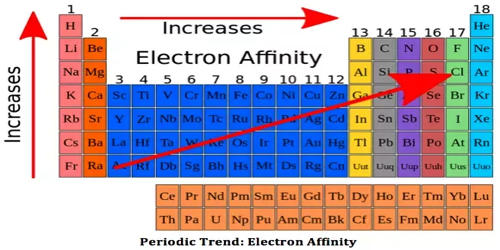# Describe on Successive Electron Affinities

Successive Electron Affinities: Electron affinity is the affinity of an element to an electron. As more than one electron can be introduced in an atom, it is called second electron affinity for the addition of two electrons and so on. The first Electron Affinities of active non-metals is positive (exothermic) while the second E. A. even for the formation of oxide or sulphide ion is negative (endothermic). For example, the overall E.A. for the formation of oxide or sulphide ions has been found to be endothermic to the extent of 640 and 390 kJ mol-1 respectively. This is measured by the energy released when an element in its gaseous state accepts an electron to form an anion.

X (g) + e + energy → X2-(g)

It is interesting to note that the electron affinity of elements having a d10 s2 configuration has been found to be negative. This is so due to the accommodation of the electron in the higher p-orbital (Zn = -87 kJ mol-1, Cd = -56 kJ mol-1).

Order of successive electron affinity

The second electron affinity (EA2) is always larger than the first electron affinity (EA1) as it is hard to add an electron into a negative ion than a neutral atom. For illustration, the overall E.A. for the formation of oxide or sulphide ions has been determining to be endothermic to the extent of 640 and 390 kJ mol-1 correspondingly.

X (g) + e + energy → X2- (g)

As for example the electron affinity of oxygen to add two electrons are:

• O (g) + e → O (g)     EA1 = -142 Kj mol-1
• O (g) + e → O2- (g)    EA2 = + 844 Kj mol-1

Here we can see that the second electron affinity is much higher than the first one. Thus in general successive electron affinity increase in magnitude EA1 < EA2 < EA3 and so on. It is interesting to observe that the electron affinity of elements having d10 s2 configuration has been found out to be negative. This is consequently due to the accommodation of the electron in the higher p-orbital (i.e., Zn = -87 kJ mol-1, Cd = -56 kJ mol-1).

Elements of group 17 possess a high electron affinity. The reason for this is that by picking up an electron halogen attain the stable noble gas electronic configuration.

The electron affinity is expressed in kJ mol-1. Electron affinity is defined as the change in energy (in kJ/mole) of a neutral atom when an electron is added to the atom to form a negative ion.